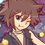# Construction of anything from any given length.

Given a segment length $$a$$ and $$b$$, we can't construct $$a^{2}$$, $$b^{2}$$, or $$a^{2} + b^{2}$$, but we can do $$\sqrt{a^{2}+b^{2}}$$ by Pythagoras.

We can't construct $$\sqrt{a}$$, $$\sqrt{b}$$, but we can do $$\sqrt{ab}$$ by drawing semicircle on $$a+b$$ and draw a line perpendicular to the point these 2 segments intersect. The line from circumference and the intersection has the length $$\sqrt{ab}$$

What I see is that the degree of what we want to construct tells you if it can be constructed or not. Degree is the exponent of any length. It can be constructed iff the degree of given length is equal to degree of length we want to construct. (Note: the description is not fully written yet, I have too much homework to do XD.)

For example, given length $$a$$, $$b$$ have degree $$1$$, but $$a^{2}$$ has degree $$2$$, and also $$a^{2} + b^{2}$$ (like polynomials, adding polynomials only consider the highest degree of result). And then, $$\sqrt{a^{2}+b^{2}}$$ has degree 1. So we can construct it.

Another example, given length $$a$$, $$b$$ have degree $$1$$, $$\sqrt{a}$$, $$\sqrt{b}$$ have degree $$\frac{1}{2}$$ because $$\sqrt{a} = a^{\frac{1}{2}}$$. But $$\sqrt{ab}$$ has degree $$\frac{1}{2} + \frac{1}{2} = 1$$, so we can construct it.

Are there any proofs or, if you wish, counterexamples of this thing I don't even have an idea what to call. It just popped out of my mind yesterday. XD

Maybe the degree is the invariance of construction? I don't know. :3Note by Samuraiwarm Tsunayoshi
4 years, 10 months ago

MarkdownAppears as
*italics* or _italics_ italics
**bold** or __bold__ bold
- bulleted- list
• bulleted
• list
1. numbered2. list
1. numbered
2. list
Note: you must add a full line of space before and after lists for them to show up correctly
paragraph 1paragraph 2

paragraph 1

paragraph 2

[example link](https://brilliant.org)example link
> This is a quote
This is a quote
    # I indented these lines
# 4 spaces, and now they show
# up as a code block.

print "hello world"
# I indented these lines
# 4 spaces, and now they show
# up as a code block.

print "hello world"
MathAppears as
Remember to wrap math in $$...$$ or $...$ to ensure proper formatting.
2 \times 3 $$2 \times 3$$
2^{34} $$2^{34}$$
a_{i-1} $$a_{i-1}$$
\frac{2}{3} $$\frac{2}{3}$$
\sqrt{2} $$\sqrt{2}$$
\sum_{i=1}^3 $$\sum_{i=1}^3$$
\sin \theta $$\sin \theta$$
\boxed{123} $$\boxed{123}$$

## Comments

Sort by:

Top Newest

Any geometry or construction is scalable, which is why for length measures, the degree has to be 1, for area measures, 2, etc. There is no such thing as length "1" in geometry, because what scale are we using, English or Metric, or something else?

- 4 years, 10 months ago

Log in to reply

Are there any reason why the length has to be degree 1 only? And what defines them to be degree 1?

- 4 years, 10 months ago

Log in to reply

Look at this is a matter of degrees of freedom. If you were a point, you could only roam along the length of a "length", like a train on a track, hence it just has 1 degree of freedom. A ship on the sea, on the other hand, has 2 degrees of freedom, it can roam along the length and breadth of an area. A spaceship can roam in space, with 3 degrees of freedom. In mathematics, it means that to define a point on a curve with a parametric equation, you need 1 variable. To define a point on a surface, you need 2. To define a point in a solid, 3. And so on. Now, but there has been some mathematics developed on the idea of "fractional dimensions", i.e., non-integer degrees of freedom, but that's another subject, mostly having to do with fractals, and can be shelved for another time.

- 4 years, 10 months ago

Log in to reply

To construct $$\sqrt{a}$$, just consider two segments, $$a=a$$ and $$b=1$$, and construct $$\sqrt{ab}=\sqrt{a}$$. However, if you mean using only $$a$$ and $$b$$, then maybe...

EDIT: I see. Thanks @Michael Mendrin

- 4 years, 10 months ago

Log in to reply

×

Problem Loading...

Note Loading...

Set Loading...